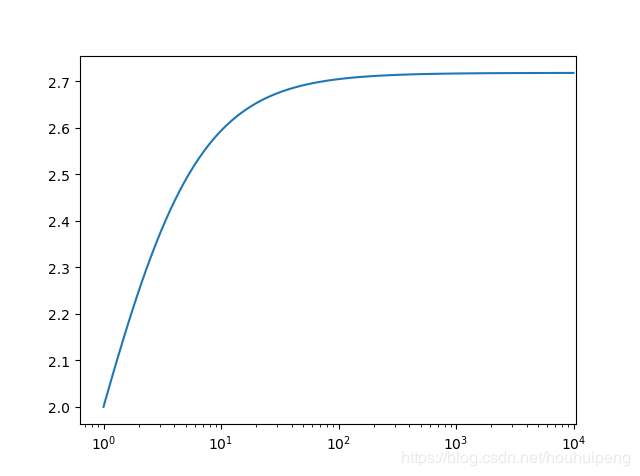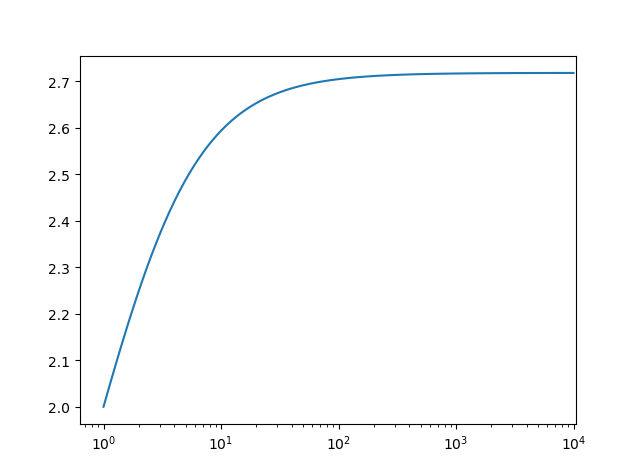• 2018-03-01 16:00:58
首先要导入 math 模块：
import math
import numpy as np

math.log(8,2)，此为以2为底8的对数
等于 math.log2(8);
等于np.log2(8)

自然对数： 以e为底的对数。
e = math.e 约等于 2.718281828459045；

x的自然对数为：
math.log(x,math.e)；
等于np.log(x)。
更多相关内容
• Python数学小实验——自然对数e的理解 先来输出一下自然对数e： >>>from math import e >>>print (e) 2.718281828459045 一个神奇的数字 import numpy as np import matplotlib.pyplot as ...

Python数学小实验——自然对数e的理解
先来输出一下自然对数e

    >>>from math import e
>>>print (e)
2.718281828459045


一个神奇的数字import numpy as np
import matplotlib.pyplot as plt

x = np.logspace(0,4,num=100,dtype=float)
y = (1+1/x)**x
plt.plot(x,y)
plt.show()复利极限的理解

    >>>k_1=1*(1+1)   #假设我有1块，年利率是100%，一年定期后，我有
>>>k_2=1*(1+1/2)**2   #存两个半年
>>>k_365=1*(1+1/365)**365   #存365天
>>>print(k_1)
2
>>print(k_2)
2.25
>>print(k_365)
2.7145674820219727理解e是复利增长的极限，有1块钱，复利100%，不管分成多少次，本利和都不会超过e≈2.718

从泰勒展开式来看
泰勒展开式：

f(x)=f(x0)+f′(x0)(x−x0)+12f’’(x0)(x−x0)2+…+f(n)(x−x0)nn!+Rn(x)
f(x)=f(x_0)+f’(x_0)(x-x_0)+\frac{1}{2}f’’(x_0)(x-x_0)2+…+\frac{f{(n)}(x-x_0)^n}{n!}+R_n(x)
f(x)=f(x 0 ​ )+f ′ (x 0 ​ )(x−x 0 ​ )+ 2 1 ​ f ′′ (x
0 ​ )(x−x 0 ​ ) 2 +…+ n! f (n) (x−x 0 ​ ) n ​ +R
n ​ (x)

其中n阶泰勒余项 Rn(x)=f(n+1)(ξ)(n+1)!(x−x0)n+1
R_n(x)=\frac{f{(n+1)}(\xi)}{(n+1)!}(x-x_0){n+1} R n ​ (x)=
(n+1)! f (n+1) (ξ) ​ (x−x 0 ​ ) n+1

令f(x)=ex,x=1,x0=0 f(x)=e^x,x=1,x_0=0f(x)=e x ,x=1,x 0 ​ =0可得：
e=1+11!+12!+…+1n!+R(n)
e=1+\frac{1}{1!}+\frac{1}{2!}+…+\frac{1}{n!}+R(n) e=1+ 1! 1 ​ +
2! 1 ​ +…+ n! 1 +R(n)

    def factorial(n):
result = 1
for i in range(1,n+1):
result *= i
return 1/result

ee=1 for i in range(1,10):
ee += factorial(i) print(ee)


计算到第10项，可得e=2.7182815255731922，已经非常吻合。

## 可进一步了解：https://en.wikipedia.org/wiki/E_(mathematical_constant)

展开全文程序员 新手入门
• Python数学小实验（1）——自然对数e的理解 先来输出一下自然对数e： >>>from math import e >>>print (e) 2.718281828459045 一个神奇的数字 import numpy as np import matplotlib....

# Python数学小实验（1）——自然对数e的理解

先来输出一下自然对数e：

>>>from math import e
>>>print (e)
2.718281828459045


## 一个神奇的数字

import numpy as np
import matplotlib.pyplot as plt

x = np.logspace(0,4,num=100,dtype=float)
y = (1+1/x)**x
plt.plot(x,y)
plt.show()## 复利极限的理解

>>>k_1=1*(1+1)   #假设我有1块，年利率是100%，一年定期后，我有
>>>k_2=1*(1+1/2)**2   #存两个半年
>>>k_365=1*(1+1/365)**365   #存365天
>>>print(k_1)
2
>>print(k_2)
2.25
>>print(k_365)
2.7145674820219727


理解e是复利增长的极限，有1块钱，复利100%，不管分成多少次，本利和都不会超过e≈2.718

## 从泰勒展开式来看

泰勒展开式： f ( x ) = f ( x 0 ) + f ′ ( x 0 ) ( x − x 0 ) + 1 2 f ′ ′ ( x 0 ) ( x − x 0 ) 2 + . . . + f ( n ) ( x − x 0 ) n n ! + R n ( x ) f(x)=f(x_0)+f&#x27;(x_0)(x-x_0)+\frac{1}{2}f&#x27;&#x27;(x_0)(x-x_0)^2+...+\frac{f^{(n)}(x-x_0)^n}{n!}+R_n(x)
其中n阶泰勒余项 R n ( x ) = f ( n + 1 ) ( ξ ) ( n + 1 ) ! ( x − x 0 ) n + 1 R_n(x)=\frac{f^{(n+1)}(\xi)}{(n+1)!}(x-x_0)^{n+1}

f ( x ) = e x , x = 1 , x 0 = 0 f(x)=e^x,x=1,x_0=0 可得：
e = 1 + 1 1 ! + 1 2 ! + . . . + 1 n ! + R ( n ) e=1+\frac{1}{1!}+\frac{1}{2!}+...+\frac{1}{n!}+R(n)

def factorial(n):
result = 1
for i in range(1,n+1):
result *= i
return 1/result

ee=1
for i in range(1,10):
ee += factorial(i)
print(ee)


计算到第10项，可得e=2.7182815255731922，已经非常吻合。

展开全文• e为底的对数(自然对数) np.log10(x) 以10为底的对数 np.log2(x) 以2为底的对数 np.log1p(x) 等价于：np.log(x + 1) 备注：np.expm1(x) 等价于 np.exp(x) – 1，也是np.log1p(x)的逆运算。 1.2 求以任意数...
• ## pythone怎么表示

万次阅读 2020-12-04 01:34:12
语法以下是 exp() 方法的语法:importmathmath.exp(x)相关推荐：《Python教程》注意：exp()是不能直接访问的，需要导入 math 模块，通过静态对象调用该方法。参数x -- 数值表达式。返回值返回x的指数，ex。实例以下...exp()方法返回x的指数，ex。

语法

以下是 exp() 方法的语法:import math

math.exp( x )

相关推荐：《Python教程》

注意：exp()是不能直接访问的，需要导入 math 模块，通过静态对象调用该方法。

参数

x -- 数值表达式。

返回值

返回x的指数，ex。

实例

以下展示了使用 exp() 方法的实例：#!/usr/bin/python

import math   # 导入 math 模块

print "math.exp(-45.17) : ", math.exp(-45.17)

print "math.exp(100.12) : ", math.exp(100.12)

print "math.exp(100.72) : ", math.exp(100.72)

print "math.exp(119L) : ", math.exp(119L)

print "math.exp(math.pi) : ", math.exp(math.pi)

以上实例运行后输出结果为：math.exp(-45.17) :  2.41500621326e-20

math.exp(100.12) :  3.03084361407e+43

math.exp(100.72) :  5.52255713025e+43

math.exp(119L) :  4.7978133273e+51

math.exp(math.pi) :  23.1406926328

展开全文• 详细内容log() 返回 x 的自然对数。math.log(x) 就相当于数学中的ln(x)，x>0，求底数为e的对数，e = 2.718281828459；math.log10(x) 就相当于数学中的lg(x)，x>0，求底数为10的对数。可以通过log(x[, base])来...
• 引言我们知道，对数函数 ln(x) 可以展开为泰勒级数：但是下面这个泰勒级数展开式收敛得更快：经过简单计算可知上式中 y = (x - 1) / (x + 1) 。实现该算法的 C# 程序根据上面的第二个泰勒级数展开式，我们可以为 C# ...
• ## python求对数

千次阅读 2019-04-10 14:24:54
此处先介绍log常用的两个底数计算（以10为底和以e为底），以后再补充完毕 1以10为底： import numpy as np np.log10(x)12 ...log下什么都不写默认是自然对数 如： >>> np.log(np.e) 1.0 >>...
• ## Python 求对数

千次阅读 2019-09-18 15:58:58
log() 返回 x 的自然对数。 2. 语法 以下是 log() 方法的语法: import math math.log(x[, base]) 注意：log()是不能直接访问的，需要导入 math 模块，通过静态对象调用该方法。 3. 参数 x – 数值...
• 循环结构是编程中经常使用的语法，循环结构的巧妙使用对编程来说很重要。刚才群里还有同学说自己不会用循环，其实很简单，只是没有用过才觉得难。这里介绍Python循环语法的用法...
• 最近在调代码，碰到幂函数、指数函数，总是提示ValueError: math domain errorValueError: negative number cannot be raised to a fractional power这些异常、错误，发现里边有很多细节问题：python中，想要表示a的...
• python对数函数Logarithms are used to depict and represent large numbers. The log is an inverse of the exponent. This article will dive into the Python log() functions. The logarithmic functions of ...
• 在数学运算中，如果没有计算器，对于很大的数字相乘，我们...而在python中，也有计算对数的方法，那就是对数函数log函数。本文将向大家介绍log函数的表述语句、参数和返回值，并以实例演示用log函数计算对数的过程。...
• ## python中取对数

千次阅读 2020-12-19 19:30:38
技术 | Python从零开始系列连载(二十七)我们接着上一期的Python，继续跟大家分享有关Python中常用的数值计算和正则表达式。运算符1)数值运算+、-、*、/% (求余数)// (注意Python中的整除是floor除，即向下除法)**2)...
• python高精度求自然常数e泰勒展开与e的求法python和神奇的decimal计算比较完整代码 泰勒展开与e的求法 大家伙儿知道计算机里的eee是怎么求出来的吗? 这还要从神奇的泰勒展开讲起 …… 简单的说，就是eee，可以表示...数学 经验分享
• 【问题】有这样的字符串，是以e为底的，自然对数值：2.1690427e-005 -1.1694737e-003 -6.1193734e-0048.9455025e-004 -8.6277081e-004 -7.2735757e-004想要将每个值，都转换为对应的浮点数值。【解决过程】1.参考：...
• e的展开式e的近似值e ≈ 2.71828 18284 59045 23536 02874 71352 66249 77572 47093 69995 95749 66967 62772 40766 30353 54759 45713 82178 52516 64274 27466 39193 20030 59921 81741 35966 29043 57290 03342 ...
• 目录Math模块pi数字常量，圆周率e表示一个常量sqrt(x)求x的平方根fabs(x) 返回x的绝对值factorial(x)取x的阶乘的值fmod(x,y)得到x/y的余数，其值是一个浮点数pow(x, y)返回x的y次方，即x**yisfinite(x) ...
• python matlibplot 画图控制对数坐标刻度显示已经通过 ax.xaxis.set_major_locator(ticker.LogLocator(base=10.0, su你试试下面这句指令，主要是subsy控制的，你的那个指令是控制x轴的，并且不太好使。我试了下面这......